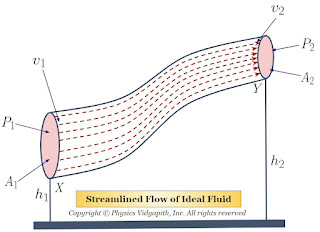## Particle Physics Basics

Particle physics is a branch of physics that studies fundamental particles and their interactions. It aims to understand the fundamental nature of matter and the universe at the smallest scales. In this article, we will discuss particle physics in detail, including its history, theoretical framework, experimental techniques, and some of its important discoveries.

History of Particle Physics:

Particle physics emerged as a distinct field of study in the early 20th century, following the discovery of the electron in 1897. In the 1930s, physicists discovered the existence of several new particles, including the muon and the pion. These discoveries led to the development of a new theory called the "particle zoo," which attempted to classify all known particles.

In the 1950s and 1960s, physicists began to develop a theoretical framework called the Standard Model, which explained the properties and interactions of known particles. This framework was based on the idea of symmetries and conservation laws, such as the conservation of electric charge and conservation of energy.

Theoretical Framework:

The Standard Model of particle physics is the theoretical framework that explains the properties and interactions of known particles. It is a quantum field theory, which means it describes particles as excitations of quantum fields that permeate space.

The Standard Model is based on the concept of fundamental particles, which are particles that cannot be broken down into smaller particles. There are mainly two different types of fundamental particles: fermions and bosons.

Fermions are particles that makeup matter, such as electrons and quarks. There are six different types of quarks, which are the building blocks of protons and neutrons. There are also six types of leptons, which include the electron and its two heavier cousins, the muon and the tau.

Bosons are particles that mediate the fundamental forces, such as the photon for the electromagnetic force and the W and Z bosons for the weak force. The strong force is mediated by gluons, which are also bosons.

The Standard Model also includes the Higgs boson, which gives mass to the other particles. The Higgs boson was discovered in 2012 at the Large Hadron Collider (LHC) in Geneva, Switzerland.

Experimental Techniques:

Particle physics experiments require high-energy particle accelerators to create and study the particles. The most powerful particle accelerator currently in operation is the LHC, which is a circular accelerator located 100 meters underground near Geneva, Switzerland.

The LHC collides protons or heavy ions at extremely high energies, producing a spray of particles that are detected by massive detectors surrounding the collision points. The detectors are designed to measure the properties of the particles, including their energy, momentum, and charge.

Other experimental techniques used in particle physics include cosmic ray experiments, which study particles that arrive from outer space, and neutrino experiments, which study the elusive neutrino particle.

Important Discoveries:

Particle physics has led to many important discoveries that have transformed our understanding of the universe. There are some of the most significant discoveries include:

1. Discovery of the electron and its properties, including its charge and mass.
2. Discovery of the proton and neutron and their properties, including their charge and mass.
3. Development of the theory of quantum electrodynamics (QED), which explains the behavior of charged particles and the electromagnetic force.
4. Discovery of the weak force and the W and Z bosons that mediate it.
5. Discovery of the Higgs boson, which gives mass to the other particles.
6. Confirmation of the existence of neutrinos and their properties, including their mass.
Conclusion:

Particle physics is a fascinating field of study that seeks to understand the fundamental nature of matter and the universe. The Standard Model provides a theoretical framework that explains the properties and interactions of the known particles, while experimental.

## Popular Posts

### Bernoulli's Theorem and Derivation of Bernoulli's EquationStatement of Bernoulli's Theorem: When an ideal fluid (i.e incompressible and non-viscous Liquid or Gas) flows in streamlined motion from one place to another, then the total energy per unit volume (i.e Pressure energy + Kinetic Energy + Potential Energy) at each and every of its path is constant. $P+\frac{1}{2}\rho v^{2} + \rho gh= constant$ Derivation of Bernoulli's Theorem Equation: Let us consider that an incompressible and non-viscous liquid is flowing in streamlined motion through a tube $XY$ of the non-uniform cross-section. Now Consider: The Area of cross-section $X$ = $A_{1}$ The Area of cross-section $Y$ = $A_{2}$ The velocity per second (i.e. equal to distance) of fluid at cross-section $X$ = $v_{1}$ The velocity per second (i.e. equal to distance) of fluid at cross-section $Y$ = $v_{2}$ The Pressure of fluid at cross-section $X$ = $P_{1}$ The Pressure of fluid at cross-section $Y$ = $P_{2}$ The height of cross-section $X$ from